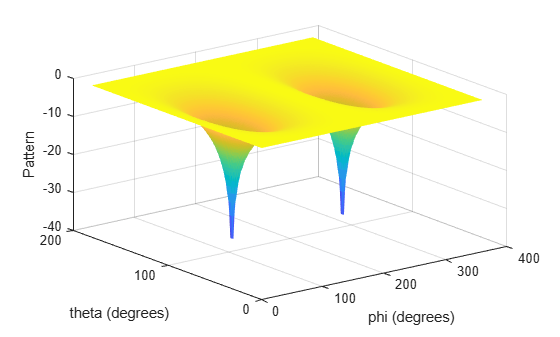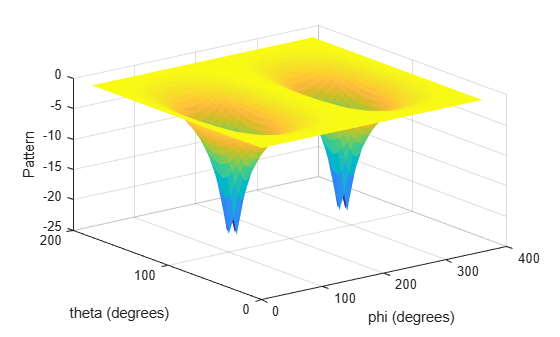Documentation

### This is machine translation

Mouseover text to see original. Click the button below to return to the English version of the page.

# azel2phithetapat

Convert radiation pattern from azimuth-elevation to phi-theta coordinates

## Syntax

``pat_phitheta = azel2phithetapat(pat_azel,az,el)``
``pat_phitheta = azel2phithetapat(pat_azel,az,el,phi,theta)``
``[pat_phitheta,phi_pat,theta_pat] = azel2phithetapat(___)``

## Description

example

````pat_phitheta = azel2phithetapat(pat_azel,az,el)` expresses the antenna radiation pattern `pat_azel` in φ/θ angle coordinates instead of azimuth/elevation angle coordinates. `pat_azel` samples the pattern at azimuth angles in `az` and elevation angles in `el`. The `pat_phitheta` matrix covers φ values from 0 to 180 degrees and θ values from 0 to 360 degrees. `pat_phitheta` is uniformly sampled with a step size of 1 for φ and θ. The function interpolates to estimate the response of the antenna at a given direction.```

example

````pat_phitheta = azel2phithetapat(pat_azel,az,el,phi,theta)` uses vectors `phi` and `theta` to specify the grid at which to sample `pat_phitheta`. To avoid interpolation errors, `phi` should cover the range [0, 180], and `theta` should cover the range [0, 360].```

example

````[pat_phitheta,phi_pat,theta_pat] = azel2phithetapat(___)`returns vectors containing the φ and θ angles at which `pat_phitheta` samples the pattern, using any of the input arguments in the previous syntaxes.```

## Examples

collapse all

Convert a radiation pattern to φ/θ form, with the φ and θ angles spaced 1 degree apart.

Define the pattern in terms of azimuth and elevation.

```az = -180:180; el = -90:90; pat_azel = mag2db(repmat(cosd(el)',1,numel(az)));```

Convert the pattern to φ/θ space.

`pat_phitheta = azel2phithetapat(pat_azel,az,el);`

Plot the result of converting a radiation pattern to $\varphi /\theta$ space with the $\varphi$ and $\theta$ angles spaced 1 degree apart.

The radiation pattern is the cosine of the elevation.

```az = -180:180; el = -90:90; pat_azel = repmat(cosd(el)',1,numel(az));```

Convert the pattern to $\varphi /\theta$ space. Use the returned $\varphi$ and $\theta$ angles for plotting.

`[pat_phitheta,phi,theta] = azel2phithetapat(pat_azel,az,el);`

Plot the result.

```H = surf(phi,theta,mag2db(pat_phitheta)); H.LineStyle = 'none'; xlabel('phi (degrees)'); ylabel('theta (degrees)'); zlabel('Pattern');```Convert a radiation pattern to $\varphi /\theta$ space with $\varphi$ and $\theta$ angles spaced 5 degrees apart.

The radiation pattern is the cosine of the elevation.

```az = -180:180; el = -90:90; pat_azel = repmat(cosd(el)',1,numel(az));```

Define the set of $\varphi$ and $\theta$ angles at which to sample the pattern. Then, convert the pattern.

```phi = 0:5:360; theta = 0:5:180; pat_phitheta = azel2phithetapat(pat_azel,az,el,phi,theta);```

Plot the result.

```H = surf(phi,theta,mag2db(pat_phitheta)); H.LineStyle = 'none'; xlabel('phi (degrees)'); ylabel('theta (degrees)'); zlabel('Pattern');```## Input Arguments

collapse all

Antenna radiation pattern in azimuth/elevation form, specified as a Q-by-P matrix. `pat_azel` samples the 3-D magnitude pattern in decibels, in terms of azimuth and elevation angles. P is the length of the `az` vector, and Q is the length of the `el` vector.

Data Types: `double`

Azimuth angles at which `pat_azel` samples the pattern, specified as a vector of length P. Each azimuth angle is in degrees, between –180 and 180.

Data Types: `double`

Elevation angles at which `pat_azel` samples the pattern, specified as a vector of length Q. Each elevation angle is in degrees, between –90 and 90.

Data Types: `double`

Phi angles at which `pat_phitheta` samples the pattern, specified as a vector of length L. Each φ angle is in degrees, between 0 and 360.

Data Types: `double`

Theta angles at which `pat_phitheta` samples the pattern, specified as a vector of length M. Each θ angle is in degrees, between 0 and 180.

Data Types: `double`

## Output Arguments

collapse all

Antenna radiation pattern in phi/theta form, returned as an M-by-L matrix. `pat_phitheta` samples the 3-D magnitude pattern in decibels, in terms of φ and θ angles. L is the length of the `phi_pat` vector, and M is the length of the `theta` vector.

Phi angles at which `pat_phitheta` samples the pattern, returned as a vector of length L. Angles are expressed in degrees.

Theta angles at which `pat_phitheta` samples the pattern, returned as a vector of length M. Angles are expressed in degrees.

collapse all

### Azimuth Angle, Elevation Angle

The azimuth angle of a vector is the angle between the x-axis and the orthogonal projection of the vector onto the xy plane. The angle is positive in going from the x axis toward the y axis. Azimuth angles lie between –180 and 180 degrees. The elevation angle is the angle between the vector and its orthogonal projection onto the xy-plane. The angle is positive when going toward the positive z-axis from the xy plane. These definitions assume the boresight direction is the positive x-axis.

### Note

The elevation angle is sometimes defined in the literature as the angle a vector makes with the positive z-axis. The MATLAB® and Phased Array System Toolbox™ products do not use this definition.

This figure illustrates the azimuth angle and elevation angle for a vector that appears as a green solid line. The coordinate system is relative to the center of a uniform linear array, whose elements appear as blue circles.### Phi Angle, Theta Angle

The φ angle is the angle from the positive y-axis toward the positive z-axis, to the vector’s orthogonal projection onto the yz plane. The φ angle is between 0 and 360 degrees. The θ angle is the angle from the x-axis toward the yz plane, to the vector itself. The θ angle is between 0 and 180 degrees.

The figure illustrates φ and θ for a vector that appears as a green solid line. The coordinate system is relative to the center of a uniform linear array, whose elements appear as blue circles.The coordinate transformations between φ/θ and az/el are described by the following equations

`$\begin{array}{l}\mathrm{sin}\left(\text{el}\right)=\mathrm{sin}\varphi \mathrm{sin}\theta \hfill \\ \mathrm{tan}\left(\text{az}\right)=\mathrm{cos}\varphi \mathrm{tan}\theta \hfill \\ \hfill \\ \mathrm{cos}\theta =\mathrm{cos}\left(\text{el}\right)\mathrm{cos}\left(\text{az}\right)\hfill \\ \mathrm{tan}\varphi =\mathrm{tan}\left(\text{el}\right)/\mathrm{sin}\left(\text{az}\right)\hfill \end{array}$`